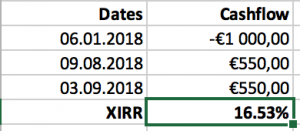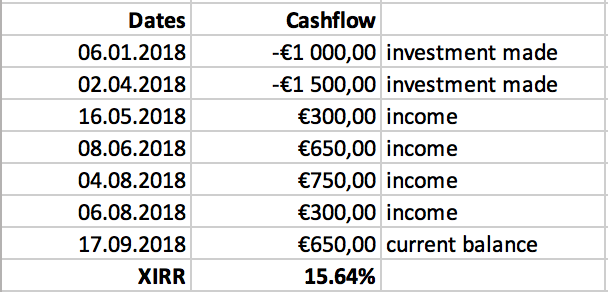# The expected rate of return and XIRR## What does the expected rate of return mean?

Cash flows received from crowdfunding projects can vary greatly – for example, proceeds from equity capital investments depend on the success of the implementation of the business plan. In the case of an apartment building in development, overly slow sales and increasing input prices may constitute a risk. The opposite may also occur: the input prices are stable, but the market situation allows selling apartments faster than expected despite increasing sales prices.

In the case of business loans with a set payment schedule, there is slightly more clarity, but possible days of late payment interest must be considered – they may both increase and decrease the rate of return. It all depends on whether the late payment interest rate is the same as the interest rate of the loan or differs from it.

In both cases, investors are interested in the expected rate of return because based on that, decisions can be made about investing capital. Crowdestate uses the XIRR function, known from spreadsheet programs, to calculate the expected rate of return. As sums received from the project may differ in size and frequency, the XIRR function calculates the internal rate of return for an irregular cash flow. Here is an example.

Let us assume that we invest 1,000 euros to an equity capital project on 6 January. The market is more active than expected, which is why the developer decides to make the first disbursement of 550 euros on 9 August and the last, also of 550 euros, on 3 September.The rate of return calculated with the XIRR method is 16.53%, but in reality, we earned a 10% rate of return on the initial investment. Which number is correct?

Both calculations are correct, but they show different things. The return on investment (ROI) shows the amount of money we have earned compared to the initial investment. XIRR presumes that the investor has constant access to similar projects – in simpler terms, if the available capital can be reinvested into a project with a similar rate of return, the investor will earn 165.3 euros in interest income on 1,000 euros in one year. Therefore, XIRR considers both the time of receiving the money and the sizes of the sums, based on which, it finds an interest rate converted to an annual basis, by which the value of the investment increases. Therefore, XIRR is a discount rate that, when implemented, allows us to see the current value of all cash flows.### Loan interest and internal rate of return are different

In the case of projects with fixed interest rates, the assessment of the expected rate of return is generally quite precise. For example, in the finished L. Koidula 32 (II) project, the interest rate was 11%, but the actual rate of return was 11.05%. The Koidula project was unique due to its single disbursement, which minimized the difference between the interest rate and the actual rate of return.

When financing the operating capital of Global Nord Timber (III), the sponsor of the project asked investors for 400,000 euros for up to seven months. It was agreed that the sponsor would pay an interest of 15% from the principal amount of the loan. Monthly repayments would consist of 28,000 euros plus current accumulated interest. The last payment would be 260,000 euros plus interest. Assuming that all payments take place according to schedule, the rate of return of the project is 16.1% with the XIRR method. This is slightly higher than the agreed 15% interest rate because the XIRR method assumes we can reinvest the repaid money under the same conditions each month.

### The expected rate of return on equity capital projects

In the case of equity capital projects, Crowdestate offers three possible scenarios: a negative scenario, a base scenario, and an optimistic scenario. With all three scenarios, the cash flows of the project are assessed from a conservative, probable, and optimistic viewpoint and the expected rate of return is calculated based on these assessments.

The expected rate of return is, therefore, a function of Crowdestate’s best assessment of possible proceeds.

## Example of calculating XIRR

Here are some tips for if you wish to calculate the rate of return of your portfolio yourself using the XIRR method.

Insert the dates in the first column and the investment sums in the second. The picture below shows that investment sums can be positive or negative. The logic that is generally used states that when making an investment, we give away money, negatively affecting the amount of money we have. Therefore, these results are marked as negative. Incoming money (repayments of the principal amount, interests, and late payment interests) are marked as positive, because they increase the amount of money we have, positively affecting the sum.In order to calculate the long-term XIRR of your portfolio, you will need to mark the current date and the current balance of the portfolio on the last line. As shown on the sample portfolio below, two investments have been made, due to which, four repayments have been made with 650 euros still to be received. If this sum were received on 17 September 2018, the internal rate of return of this portfolio would be 15.64%. However, every additional day causes the internal rate of return to decrease due to the time value of money – the euro of today is worth more than the euro of tomorrow.In case you don’t have an account with Crowdestate yet, you can create it here.

Thank you for investing with us! 🙂

This site uses Akismet to reduce spam. Learn how your comment data is processed.